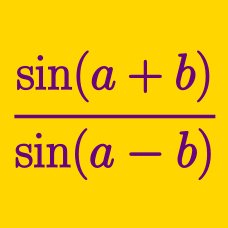Geometry

# Tangent - Sum and Difference Formulas

Which of the following is equal to

$\frac{ \tan 43 ^ \circ - \tan 14 ^ \circ } { 1 + \tan 43 ^ \circ \times \tan 14 ^ \circ } ?$

Which of the following is equal to

$\frac{ \tan 19 ^ \circ + \tan 26 ^ \circ } { 1 - \tan 19 ^ \circ \times \tan 26 ^ \circ } ?$

If $$\tan x=\frac{1}{14}$$, the value of $$\tan \left(x+\frac{\pi}{4}\right)$$ can be expressed as $$\frac{a}{b}$$, where $$a$$ and $$b$$ are coprime positive integers. What is the value of $$a+b$$?

If $$\tan N ^ \circ = \tan 12 ^ \circ + \sec 12 ^ \circ$$, where $$0 \leq N \leq 90$$, what is the value of $$N$$?

Which of the following expressions is equal to $$\tan 2 \theta$$?

×